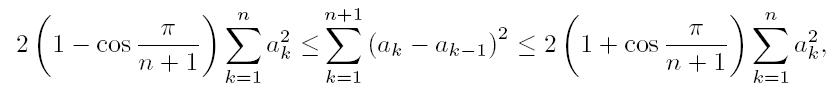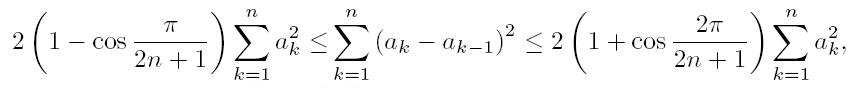The Australian Journal of Mathematical Analysis and Applications

 Home News Editors Volumes RGMIA Subscriptions Authors Contact

ISSN 1449-5910

Paper Information

Paper Title:

A Simple New Proof of Fan-Taussky-Todd Inequalities

Author(s):

Zhi-Hua Zhang and Zhen-Gang Xiao

Zixing Educational Research Section,
Chenzhou City, Hunan 423400, P. R. China.
Zhi-hua Zhang
Url: http://www.hnzxslzx.com/zzhweb/

Department Of Mathematics, Hunan Institute Of Science And Technology,
Yueyang City, Hunan 423400, P. R. China.
Zhen-gang Xiao

Abstract:

In this paper we present simple new proofs of the inequalities:which holds for all real numbers a0 = 0, a1, · · · , an, an+1 = 0 and the coefficients 2(1 - cos(π/(n + 1))) and 2(1 + cos(π/(n + 1))) are the best possible; andwhich holds for all real numbers a0 = 0, a1, · · · , an and the coefficients 2(1-cos(π/(2n + 1))) and 2(1 + cos(π/(2n + 1))) are the best possible.

Full Text PDF: